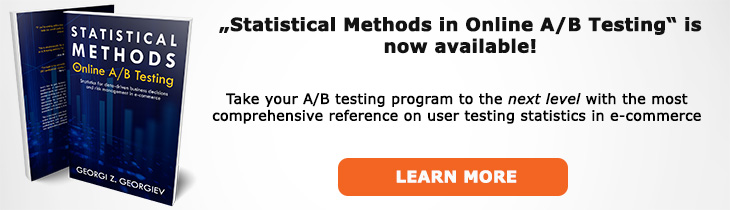# What does "One-Tailed Test" mean?

Definition of One-Tailed Test in the context of A/B testing (online controlled experiments).

## What is a One-Tailed Test?

Aliases: one-sided test

A one-tailed test in hypothesis testing is a test defined so that it matches a one-sided hypothesis. The "tail" references one half of the frequency distribution of the statistic one is using (e.g. a z score or a t score). It is in that side where you define your rejection region. However, this terminology can be misleading since a one-tailed distribution of the statistic is not necessary for testing a one-sided hypothesis. For example, the fact that the Χ2 (chi-square) distribution has no left tail does not mean one cannot perform a one-sided test with it to produce a p-value that corresponds to a one-sided null hypothesis. Therefore, the term "one-sided test" is recommended to avoid confusion.

If the distribution of the statistic is symmetrical one can easily convert the result from a two-tailed test to a one-tailed one by dividing a p-value by 2 or by considering the confidence level of an interval to be (100-(100-XX%)/2)% where XX% is the confidence level of a two-tailed interval.

Since in an A/B test the hypothesis of interest is usually one-sided we mostly use one-sided tests.

## Articles on One-Tailed Test

Like this glossary entry? For an in-depth and comprehensive reading on A/B testing stats, check out the book "Statistical Methods in Online A/B Testing" by the author of this glossary, Georgi Georgiev.## Glossary Index by Letter

Select a letter to see all A/B testing terms starting with that letter or visit the Glossary homepage to see all.